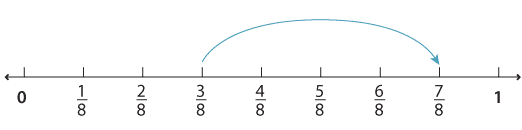Addition of fractions with the same denominator

Addition of two fractions with the same denominator can be thought of as steps on the number line.

To add $$\dfrac{3}{8} \text{and} \dfrac{4}{8} \text{we start at} \dfrac{3}{8} \text{and take a step of} \dfrac{4}{8} \text{to get} \dfrac{7}{8}$$.Detailed description

We write $$\dfrac{3}{8} + \dfrac{4}{8} = \dfrac{7}{8}$$.